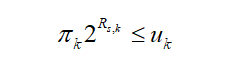# All dimensions but the one being concatenated (1) must be equal

i have error in cvx code with minimize the energy consumpution in NOMA enabled MEC system, my code is
cvx_begin
variable l1(1,K)
variable p(1,K)
variable Rt(1,K)
variable Rs(1,K)
variable phi(1,K)
variable u(1,K)
variable pai(1,K)
variable w(1,K)
variable B(K,K)
variable b(1,K)
expression m(1,K-1)
expression c1(1,K-1)
%objective function
E = zetapow_abs(c,3)pow_abs(l1,3)./0.01 + p.T;
a1 = sum(sum(B-B1+rou.
lam1));
a2 = sum(sum(B.
(1-B1)+rou.
lam2));
a3 = sum(sum(B+B’- ones(K) + eye(K) +rou.* lam3));
fun = sum(E) - 0.5rou(a1 + a2 - a3);
minimize (fun)

``````        subject to
for k = 1:K
(L(i) - l1(k))-10^5* Rs(k)<=0;
Rt(k) - log(1+b(k))<=0;
b_old(k)*pai_old(k) + pai_old(k)*(b(k)-b_old(k))+b_old(k)*(pai(k)-pai_old(k))- gamma(k)*p(k)<=0;
exp(-phi(k)*pow_abs(d,alpha)) <= epsilon;
pai_old(k)*2^Rs_old(k)+2^Rs_old(k)*(pai(k)-pai_old(k))+pai_old(k)*2^Rs_old(k)*log(2)*(2^Rs(k)-2^Rs_old(k))-u(k)<=0;
p_old(k)*u_old(k) + u_old(k)*(p(k)-p_old(k))+p_old(k)*(u(k)-u_old(k))- w(k)<=0;
phi_old(k)*w_old(k) + w_old(k)*(phi(k)-phi_old(k))+phi_old(k)*(w(k)-w_old(k))- (pai(k)+gamma(k)*p(k)-u(k))*10^-7<=0;
for l = 1:K
if l~=k
m(l) = (B_old(k,l)-gamma(l).*p_old(l))*(B(k,l)-gamma(l).*p(l))*0.25-square(B_old(k,l)-gamma(l).*p_old(l))*0.125;
c1(l) = B(k,l)-p(l);
elseif  B_old(k,l)~=0
gamma(l)*(B_old(k,l)*p_old(k) + p_old(k)*(B(k,l)-B_old(k))+B_old(k)*(p(k)-p_old(k)))<=gamma(k).* p(k);
end
end
norm([sum(m)+(pai(k)-1)*0.5 -0.5;c1(1)*0.5;c1(2)*0.5])<=(pai(k)-1)*0.5+0.5+sum(m);
end

cvx_end
``````

there is an error in NOMA decoding order

All dimensions but the one being concatenated (1) must be equal.

y = cat( 1, varargin{:} );

norm([sum(m)+(pai(k)-1)*0.5
-0.5;c1(1)*0.5;c1(2)*0.5])<=(pai(k)-1)*0.5+0.5+sum(m);

how to solve the problem?

Just before the line producing the error is reached, type one element, per command line, of the norm argument, and hit Enter. That should show you the dimensions of each element. They need to be conformal per the standard MATLAB rules so that they can be concatenated into the argument for norm.

i used this method , the elements all the scalar
cvx real affine expression (scalar)
but still has the error

Can you choose a small value of K and post a complete reproducible problem?

i’m sorry i didn’t see the message until now. i want to solve the decoding order in NOMA system. now the c1 vector is 1×3, but one element in c1 is cvx zero expression (scalar). how to remove the zero element and make c1 be a 1×2 vector?
and if the c1(1) is the zero element, can be such an error like All dimensions but the one being concatenated (1) must be equal?

If `c1` is 1 by 3, then `c1(2:3)` is 1 by 2 and has “eliminated” `c1(1)`. Does that answer your question? This works whether `c1` is a MATLAB variable, a CVX variable, or a CVX expression.

thanks professor， i solved this problem。but there is another error。

Disciplined convex programming error:
Invalid constraint: {concave} <= {constant}

b = newcnstr( evalin( ‘caller’, ‘cvx_problem’, ‘[]’ ), x, y, ‘<=’ );

pai_old(k)2^Rs_old(k)+2^Rs_old(k)(pai(k)-pai_old(k))+pai_old(k)*2^Rs_old(k)log(2)(2^Rs(k)-2^Rs_old(k))-u(k)<=0;

i debug it find that it is correct in first two loops， but when doing the third loop the code has the error.

this constraint isthen i use SCA method so the constraint rewriten as

I presume the sign of some coefficient(s) must change to make the LHS concave instead of coovex in the 3rd loop. You should examine the values of the coefficients in each loop. perhaps you need some type of safeguard to prevent this. This kind of thing (unsafeguarded changes to variables from one iteration to the next) is one of the reasons I am not a big fan of crude SCA methods instead of using a high quality safeguarded non-convex nonlinear optimizer.

For the record, I’m not a professor. Just what we call in the U.S., an ordinary Joe

thank you very much, i will examine the value carefully.